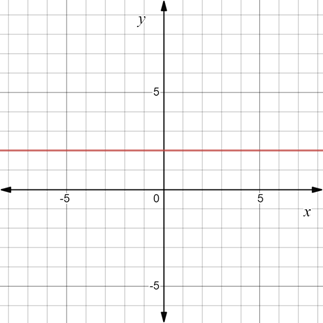# To graph : The region provided by the set { ( x , y ) | y = 2 } .### Precalculus: Mathematics for Calcu...

6th Edition
Stewart + 5 others
Publisher: Cengage Learning
ISBN: 9780840068071### Precalculus: Mathematics for Calcu...

6th Edition
Stewart + 5 others
Publisher: Cengage Learning
ISBN: 9780840068071

#### Solutions

Chapter 1.8, Problem 25E
To determine

## To graph: The region provided by the set {(x,y)|y=2} .

Expert Solution

### Explanation of Solution

Given information:

The set, {(x,y)|y=2} .

Graph:

The graph of the region provided by the set {(x,y)|y=2} is sketched in the coordinate plane.

Construct a horizontal line y=2 .

The region obtained is provided below,Interpretation:

The region provided by the set {(x,y)|y=2} is the dark red line. All the points that lie in the region have y-coordinate as 2 for all x-coordinates. The line is a horizontal line two units above the x-axis.

### Have a homework question?

Subscribe to bartleby learn! Ask subject matter experts 30 homework questions each month. Plus, you’ll have access to millions of step-by-step textbook answers!